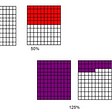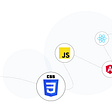`let x = z^2 in    if cond     then x+y     else 1`

In the above code x is used only in then branch and not it else branch, and which branch is chosen depends on cond. So, it is totally unnecessary and waste of resources to evaluate x beforehand. It is evaluated only when the then branch is evaluated. The following code shows exactly when it is evaluated lazily.

`let x = z^2 in    if cond     then z^2+y     else 1`

Haskell uses lazy evaluation, i.e. arguments to a function are evaluated only when they are actually used. This is exactly opposite to strict evaluation. In strict evaluation, function arguments are fully evaluated before passing them to the function. Lazy evaluation can be thought of as call-by-name evaluation in lambda world. In Haskell, the unevaluated parameter to a function is called the “thunk”.

Lazy evaluation saves memory if memory allocation is done lazily. Means, memory is allocated only when something is stored in memory not when the allocation is declared (e.g. malloc in C). But at the same time, with lazy evaluation, it is hard to predict how much memory the program is going to use throughout the program.

`foldl :: (b -> a -> b) -> b -> t a -> bfoldl _ z []     = zfoldl f z (x:xs) = foldl f (f z x) xs `

If we evaluate `foldl (+) 0 [1,2,3]` , it takes the following steps.

`foldl (+) 0 [1,2,3]    = foldl (+) (0+1) [2,3]    = foldl (+) ((0+1)+2)     = foldl (+) (((0+1)+2)+3) []    = (((0+1)+2)+3)    = ((1+2)+3)    = (3+3)    = 6`

The value of the accumulator is not required until the end. Thus we get the thunk`(((0+1)+2)+3)`. After which it gets evaluated only when answer is required to be printed on the screen. The problem with this is in every time the program needs to remember a number. With a very large list `(...(((0+1)+2)+3).....)`, this can result into a stack overflow. Haskell has foldl’ function to get rid of this big thunk problem, which requires the second argument to be evaluated before every next step.

Lazy evaluation enables us to work with infinite data structures.

“Defining an infinite data structure actually only creates a thunk, which we can think of as a “seed” out of which the entire data structure can potentially grow, depending on what parts actually are used/needed.”

In the following code snippet, two lists are added element by element until one list is empty. repeat 3 creates an infinite list containing only 3.

`adds :: (Num a) => [a] ->[a] -> [a]adds [] [] = []adds [] _ = []adds _ [] = []adds (x:xs) (y:ys) = (x+y):(adds xs ys)*Main> adds [1,2] (repeat 3)[4,5]*Main> adds (repeat 4) [2,5,6][6,9,10]*Main> adds (repeat 4) (reteat 3)**stack overflow**`

The thunks are evaluated only enough so that the Haskell pattern match goes through.

`func1: [Int] -> Intfunc1 [] = 0func x::xs = x + func1 xsfunc2 : Int -> Boolfunc2 0 = Truefunc2 1= Falsefunc2 x = func2 (x-2)`

For example, in the above example if we call (func2 (func1 [1,3,6,90])), to pattern match it will be evaluated to (func2 100), and will not be evaluated to True. It will be carried forward as (func2 100), a thunk. So in a nutshell evaluation is done until it pattern matches and not more than that.

--

--

--

## More from Priyanka Mondal

Love podcasts or audiobooks? Learn on the go with our new app.

## CS373 Fall 2021: Adeet Parikh## Introduction to the REST API — Part II## How to Choose the Best Technology Stack for Mobile App Development## How to use Git & GitHub for Version Control## [IoT] Micropython with ESP-8266 through Mosquitto MQTT## Weekly update from PointPay (August 16 — August 20, 2021)## Exploring AndroidJUnitRunner filtering options## Pandemics and Protocols## What are Percentages and How To Calculate Percentages?## The Frontend Roadmap for 2022